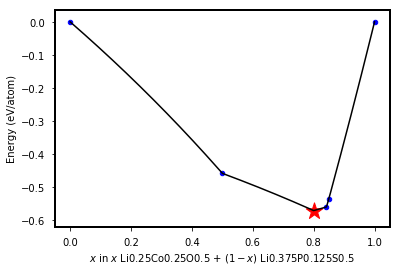# Interface Reactions¶

This notebook shows how to

• Obtain information about interface reactions between two solid substances in contact, and
• Plot reaction energy as a function of mixing ratio.

Written using:

• pymatgen==2019.2.28

We use the Materials Project API to obtain energies of compounds.

In :
from pymatgen.ext.matproj import MPRester
from pymatgen.analysis.interface_reactions import InterfacialReactivity
from pymatgen.analysis.phase_diagram import PhaseDiagram, GrandPotentialPhaseDiagram
from pymatgen import Composition, Element
%matplotlib inline

# Initialize the REST API interface. You may need to put your own API key in as an arg.
mpr = MPRester()


First, set the values of the two reactants. Optionally, simulate the case where the reaction system is in contact with an elemental reservoir.

Because the methodology here is to generate a pseudo-binary phase stability diagram of two reactants as a function of mixing ratio, the addition of an elemental reservoir implies construction of a so-called grand potential phase diagram.

In :
# Chemical formulae for two solid reactants.
reactant1 = 'LiCoO2'
reactant2 = 'Li3PS4'

# Is the system open to an elemental reservoir?
grand = True

if grand:
# Element in the elemental reservoir.
open_el = 'Co'
# Relative chemical potential vs. pure substance. Must be non-positive.
relative_mu = -1


Now, compile the critical reaction information:

In :
# Get the compositions of the reactants
comp1 = Composition(reactant1)
comp2 = Composition(reactant2)

# Gather all elements involved in the chemical system.
elements = [e.symbol for e in comp1.elements + comp2.elements]
if grand:
elements.append(open_el)
elements = list(set(elements)) # Remove duplicates

# Get all entries in the chemical system
entries = mpr.get_entries_in_chemsys(elements)

# Build a phase diagram using these entries.
pd = PhaseDiagram(entries)

# For an open system, include the grand potential phase diagram.
if grand:
# Get the chemical potential of the pure subtance.
mu = pd.get_transition_chempots(Element(open_el))
# Set the chemical potential in the elemental reservoir.
chempots = {open_el: relative_mu + mu}
# Build the grand potential phase diagram
gpd = GrandPotentialPhaseDiagram(entries, chempots)
# Create InterfacialReactivity object.
interface = InterfacialReactivity(
comp1, comp2, gpd, norm=True, include_no_mixing_energy=True, pd_non_grand=pd, use_hull_energy=False)
else:
interface = InterfacialReactivity(
comp1, comp2, pd, norm=True, include_no_mixing_energy=False, pd_non_grand=None, use_hull_energy=False)


From here, you can plot reaction energy versus mixing ratio as below. Note that the mixing ratio is between the normalized compositions of the reactants.

In :
plt = interface.plot()In :
from collections import OrderedDict
import pandas as pd

critical_rxns = [
OrderedDict([
("Atomic fraction", round(ratio, 3)),
("Reaction equation", rxn),
("E$_{rxt}$ per mol equation (kJ/mol)", round(rxn_energy, 1)),
("E$_{rxt}$ per reactant atom (eV/atom)", round(reactivity, 3)),

])
for _, ratio, reactivity, rxn, rxn_energy in interface.get_kinks()]
interface_reaction_table = pd.DataFrame(critical_rxns)
interface_reaction_table

Out:
Atomic fraction Reaction equation E$_{rxt}$ per mol equation (kJ/mol) E$_{rxt}$ per reactant atom (eV/atom)
0 0.000 Li3PS4 -> Li3PS4 0.0 0.000
1 0.500 0.3333 Li3PS4 + 0.6667 LiCoO2 -> 0.1667 Co + 0... -206.4 -0.458
2 0.800 0.1111 Li3PS4 + 0.8889 LiCoO2 -> 0.8889 Co + 0... -195.9 -0.571
3 0.842 0.08571 Li3PS4 + 0.9143 LiCoO2 -> 0.9143 Co + ... -185.3 -0.560
4 0.850 0.08108 Li3PS4 + 0.9189 LiCoO2 -> 0.8649 Co + ... -175.8 -0.535
5 1.000 LiCoO2 -> LiCoO2 -0.0 -0.000

You can also obtain the mixing ratio between the original compositions, i.e. mol fraction of the first reactant.

In :
import numpy as np
interface_reaction_table['Molar fraction'] = pd.Series(np.round(interface.get_critical_original_kink_ratio(), 3))
interface_reaction_table

Out:
Atomic fraction Reaction equation E$_{rxt}$ per mol equation (kJ/mol) E$_{rxt}$ per reactant atom (eV/atom) Molar fraction
0 0.000 Li3PS4 -> Li3PS4 0.0 0.000 0.000
1 0.500 0.3333 Li3PS4 + 0.6667 LiCoO2 -> 0.1667 Co + 0... -206.4 -0.458 0.667
2 0.800 0.1111 Li3PS4 + 0.8889 LiCoO2 -> 0.8889 Co + 0... -195.9 -0.571 0.889
3 0.842 0.08571 Li3PS4 + 0.9143 LiCoO2 -> 0.9143 Co + ... -185.3 -0.560 0.914
4 0.850 0.08108 Li3PS4 + 0.9189 LiCoO2 -> 0.8649 Co + ... -175.8 -0.535 0.919
5 1.000 LiCoO2 -> LiCoO2 -0.0 -0.000 1.000

Note that the reaction equations are Reaction objects suitable for structured analysis:

In :
rxn = critical_rxns["Reaction equation"]
print(rxn)
print(type(rxn))

0.1111 Li3PS4 + 0.8889 LiCoO2 -> 0.8889 Co + 0.3333 Li2SO4 + 0.1111 Li2S + 0.1111 Li3PO4
<class 'pymatgen.analysis.reaction_calculator.Reaction'>

In :
# Get interface reaction information for reactants LiCoO2 and Li3PS4 in open system to Co.
kinks_from_API = mpr.get_interface_reactions('LiCoO2','Li3PS4', open_el='Co', relative_mu=-1, use_hull_energy=False)

# Get inforamtion for the second critical reaction.
print(kinks_from_API)

{'ratio_atomic': 0.5000000000000001, 'ratio_molar': 0.6666666666666666, 'energy': -0.45837463535714296, 'rxn': Li3PS4 + 2 LiCoO2 -> 0.5 Co + Li2S + 1.5 CoS2 + Li3PO4, 'rxn_str': '0.333 Li3PS4 + 0.667 LiCoO2 -> 0.167 Co + 0.333 Li2S + 0.5 CoS2 + 0.333 Li3PO4', 'rxn_energy_sigdig': '-0.4584', 'rxn_energy_sigdig_kJmol': '-206.4', 'energy_per_mol_rxn_kJmol': -206.389932955848}


The critical reaction information from REST API should be the same as in the previous table:

In :
critical_rxns_from_API = [
OrderedDict([
("Atomic fraction", round(reaction['ratio_atomic'], 3)),
("Molar fraction", round(reaction['ratio_molar'], 3)),
("Reaction equation", reaction['rxn_str']),
("E$_{rxt}$ per mol equation (kJ/mol)", round(float(reaction['rxn_energy_sigdig_kJmol']),1)),
("E$_{rxt}$ per reactant atom (eV/atom)", round(reaction['energy'], 3))
])
for reaction in kinks_from_API]
pd.DataFrame(critical_rxns_from_API)

Out:
Atomic fraction Molar fraction Reaction equation E$_{rxt}$ per mol equation (kJ/mol) E$_{rxt}$ per reactant atom (eV/atom)
0 0.000 0.000 Li3PS4 -> Li3PS4 0.0 0.000
1 0.500 0.667 0.333 Li3PS4 + 0.667 LiCoO2 -> 0.167 Co + 0.33... -206.4 -0.458
2 0.800 0.889 0.111 Li3PS4 + 0.889 LiCoO2 -> 0.889 Co + 0.33... -195.9 -0.571
3 0.842 0.914 0.086 Li3PS4 + 0.914 LiCoO2 -> 0.914 Co + 0.11... -185.3 -0.560
4 0.850 0.919 0.081 Li3PS4 + 0.919 LiCoO2 -> 0.865 Co + 0.05... -175.8 -0.535
5 1.000 1.000 LiCoO2 -> LiCoO2 -0.0 -0.000